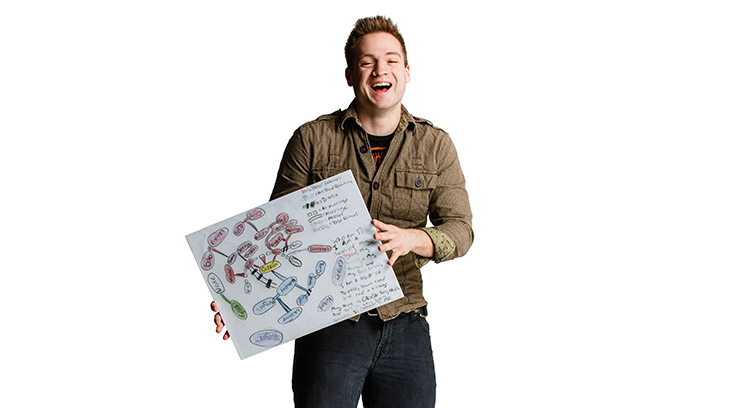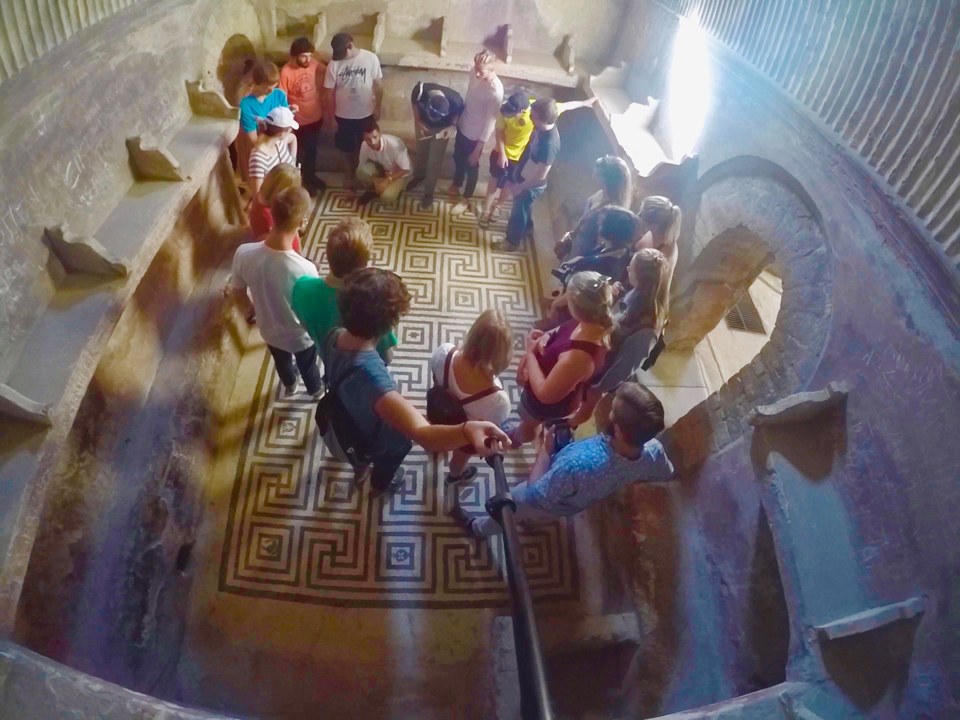# Mathematics Through the Lens of Biology

Until recently, many biologists also viewed themselves as pure experimentalists with no need for mathematics in their field, but advances in experimental techniques have generated huge amounts of data, and without mathematical modeling and analysis it would be impossible for biology to make sense of all these data.

You may recall the “application sections” in your introductory mathematics courses: velocity and acceleration, compound interest, force balance, center of mass. Most of the applications you likely encountered were from the fields of physics, engineering and economics. Except for population growth, it is unlikely that any applications in your math courses came from biology. Until recently, many biologists also viewed themselves as pure experimentalists with no need for mathematics in their field, but advances in experimental techniques have generated huge amounts of data, and without mathematical modeling and analysis it would be impossible for biology to make sense of all these data.

Last year, I taught a section of Applied Math and Modeling with a focus on mathematical biology. Often when students learn and interpret mathematics through the lens of an application, the mathematics becomes more intuitive and meaningful. My goal with this course was, first and foremost, to teach students how to write accurate, yet tractable, mathematical models. A mathematical model describes some phenomenon from the real world in the form of a mathematical formula or equation. Once the model is formulated, it can be analyzed using a huge variety of techniques, depending on the type of model and what information we want to gain from its analysis.

The second goal of my course was to introduce students to some of these analytical techniques. Because many biologists are interested in how properties change with time, many of the mathematical models in the course took the form of differential equations, which involve derivatives that describe rates of change. What do math students tend to want to do when they see an equation? Solve it; however, solving differential equations, especially those that arise in biological applications, is difficult; furthermore, while biologists are interested in changes in time, they often are interested in what happens after a long, long time. It turns out that many mathematical techniques do not require solving differential equations that allow mathematicians to determine what will happen to a system after a long, long time. Using these techniques, students were able to predict whether two interacting species that are introduced into an ecosystem (say, a predator wolf and its prey, a rabbit) will coexist after 20 years or to predict whether one, or both, of these species will become extinct. Students were able to predict how far along the California coast an initially small population of sea otters will spread, given food and environmental conditions. Students also were able to determine what the minimum susceptibility of individuals must be in order for a certain percentage of a population to die from an outbreak of severe acute respiratory syndrome. Biology provided these students with a unique way to understand the mathematical analysis methods that they were learning.

Upper-level mathematical biology courses such as mine have become more popular in undergraduate education over the past 10 years. Mathematical biology has been an active area of research for much longer, and it too has increased in popularity over the past 15 years. Mathematical biology is a huge field in itself with many different areas of application within biology. Researchers are using math to investigate topics such as how refusing vaccines for one’s children affects the probability of a measles outbreak, or how fluid flow affects the formation of biofilms, which are films of bacteria that can grow in moist places, such as rocks in streams, sewers – and showers!

Recently, I have been working on models that predict how a single biological cell interacts with its environment. Cell-environment interaction is extremely important because it affects many processes in the body, including wound healing, immune response and the development of cancer. As a result, there are huge amounts of experimental data available. Some experiments aim to determine how the cell changes its attachments to the surrounding environment, while others aim to elucidate what biochemical reactions must occur within the cell so that it attaches. Without mathematical modeling and analysis, there would be no way to begin to understand how these components all work together.

While I view mathematical biology as a rich and beautiful field, my main goal is to use this field to expose students to the power of mathematics as a tool – a tool that these students can take with them to whatever future endeavors they choose and a tool that can better help them understand the world around them.

## Next in CAS SpotlightCAS Spotlight

## More from CAS Spotlight### The CORE Semester in Rome

CAS Spotlight### Emerging Scholars

CAS Spotlight### Partnering for the Common Good

CAS SpotlightCAS Spotlight